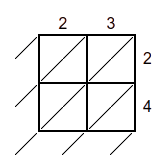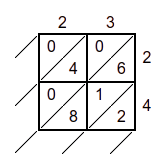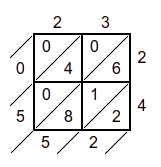# Free lattice method of multiplication worksheets

Do you want to spice your math lessons with engaging and interactive worksheets? If you answered yes, you should try our free lattice method of multiplication worksheets!

The lattice multiplication method helps multiply two or more digits using a grid or a lattice. It breaks down the multiplication process into smaller steps, making it easier for kids to understand and remember the solving process. Lattice multiplication also helps kids practice their addition and place value skills. Here, you will see how to use lattice multiplication worksheets to teach your kids how to multiply numbers from single-digit to four-digit in different combinations. You will also find some tips and tricks on how to make lattice multiplication fun and effective.

If you are looking for lattice multiplication worksheets and grids to use with your kids, you can find them here or create your own using a template.

MathSkills4Kids.com has several lattice multiplication worksheets PDF that consists of lattice multiplication worksheets for 3rd-grade, 4th-grade, and 5th-grade children with various digits combination 3 by 3, lattice multiplication worksheets 3 by 2, lattice multiplication worksheets 4 by 2 and more printable grids and templates.

• Lattice multiplication worksheets are printable sheets that have lattice grids of various sizes. Each grid has a multiplicand (the number being multiplied) and a multiplier (the number that multiplies the multiplicand) written along the top and right sides of the grid. The grid is divided into cells that have diagonal lines.

To use lattice multiplication worksheets, follow these 3 steps:

1. Write the multiplicand and the multiplieralong the top and right sides of the grid, respectively. Make sure each digit has its column or row.
2. Multiply each digit of the multiplicand by each digit of the multiplier, starting from the rightmost digits. Write the product of each pair of digits in the corresponding grid cell, with the tens digit above the diagonal line and the ones digit below the diagonal line. Write a zero above the diagonal line if the product is a single digit.
3. Add hyphens where needed: Hyphens are used to connect two-digit numbers from 21 to 99.

For example, in 2743, we write two thousand seven hundred forty-three. Note here that there is a hyphen between forty and three

4. Add up the digits along each diagonal of the grid, starting from the bottom right corner. Write the sum below each diagonal, and carry over any extra digit to the next diagonal. The final answer is read from top to bottom and left to right along the bottom and left sides of the grid.

The above steps are so fun and easy, right? Just try them out and master the skills of converting or writing numbers in words at your fingertips while uplifting your number sense, place value understanding, and spelling skills.

Here is a step-by-step lattice multiplication example; it shows how to use lattice multiplication worksheets to multiply 23 by 14:

1. Step 1. Write the multiplicand and the multiplier
2. Step 2. Multiply each digit of the multiplicand by each digit of the multiplier
3. Step 3. Add up the digits along each diagonal of the gridHere are some tips and tricks on how to make lattice multiplication more fun and effective for your kids:

• Use colorful pens or pencils to write the numbers and draw the grid. It will make the worksheet more appealing and help your kids differentiate between the digits and the lines.
• Use different shapes or symbols instead of numbers to make the worksheet more challenging and creative. For example, you can use stars, hearts, circles, squares, etc., to represent different digits. Just make sure your kids know what each shape or symbol means before they start multiplying.
• Use word problems or real-life scenarios to make the worksheet more relevant and meaningful. For example, you can ask your kids how many cookies they can bake if they have 12 trays, and each tray can hold 24 cookies. Then, use lattice multiplication to solve the problem.
• Use games or puzzles to make the worksheet more fun and interactive. For example, you can create a crossword puzzle or a bingo game using lattice multiplication problems as clues or answers.

Make these multiplication using lattice multiplication

• A) 32 x 64 = ____________

B) 97 x 17 = ____________

C) 62 x 48 = ____________

D) 21 x 37 = ____________

E) 80 x 69 = ____________

• F) 246 x 314 = ____________

G) 157 x 390 = ____________

H) 492 x 234 = ____________

I) 406 x 795 = ____________

J) 934 x 761 = ____________

• K) 3246 x 406 = ____________

L) 4573 x 1767 = ____________

M) 3954 x 5647 = ____________

N) 4915 x 2492 = ____________

O) 8374 x 25478 = ____________

•